# 5.3. MULTIPLE-USER DECENTRALIZED COMPUTER SYSTEM

## 5.2. AD HOC COMMUNICATION NETWORK

One can imagine a local network of heterogeneous computers whose communication layer is almost as good as the communication layer of the MPP architecture. Parallel programming for such networks, called in this book heterogeneous clusters, faces no specific communication-related challenges. Heterogeneous clusters are normally designed specifically for high-performance distributed computing.

However, the topology and structure of the communication network in a typical common local network of computers is determined by many different factors, among which high-performance computing is far away from being a primary factor if considered at all. The primary factors include the structure of the organization, the tasks that are solved on computers of the NoC, the security requirements, the construction restrictions, the budget limitations, the qualification of technical personnel, and so on.

An additional important factor is that the communication network is constantly developing rather than being fixed once and forever. The development is normally occasional and incremental. Therefore the structure of the communication network reflects the evolution of the organization rather than its current snapshot.

All the factors make the common communication network far away from the ideal MPP communication network, which is homogeneous with communication speedup and bandwidth being balanced with the number and speed of processors.

First of all, the common communication network is heterogeneous. The speed and bandwidth of communication links between different pairs of processors may differ significantly. Second, some of the communication links may be of low speed and/or narrow bandwidth. This makes the problem of optimal distribution of computations and communications across an NoC more difficult than across a cluster of heterogeneous processors interconnected with a homogeneous high-performance communication network. The additional difficulty comes from the larger size of the problem, which is now O(n2), where n is the total number of processors (respectively, n2 is the total number of interprocessor communication links).

Apart from that, due to low performance of some communication links, the optimal distribution of computations and communications may be across some subnetwork of the NoC, and not across the entire NoC. This substantially extends the space of possible solutions and increases the complexity of the distribution problem even further.

To take an example, consider a network of p homogeneous processors interconnected by a plain Ethernet network. The bandwidth of the Ethernet-based communication network is equal to 1 because the network cannot simultaneously carry more than one data package.

Let us estimate the time of execution of the simplest parallel algorithm of matrix-matrix multiplication from Section 4.1 on this network. Remember that the algorithm has been proved scalable when executing on an MPP. The contribution of computation in the total execution time of the parallel algorithm above will be the same:The per-processor communication cost will also be the same: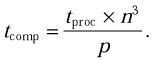However, as all of the communications are performed serially, and not in parallel, the total execution time of the parallel algorithm on the network will be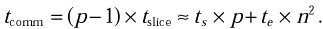The execution of the algorithm is still accelerated while upgrading the network from the one-processor to the two-processor configuration, if n is large enough (typically, if n > 200). But the algorithm is no longer scalable. Indeed, the algorithm would be scalable, if and only if ttotal were a monotonically decreasing function of p, that is, if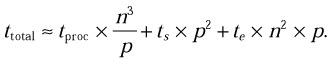or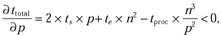This inequality will be true only if p is small enough, whatever n and the ratio between p and n are. It means that the algorithm is not scalable on the network, and there is some optimal number of processors that solves the problem the fastest. This number is very likely to be less than the total number of available processors.

But it could be just a wrong algorithm. Perhaps the improved algorithm, which is based on two-dimensional matrix decomposition, is scalable on the network. Then the total execution time of the improved algorithm on the network would be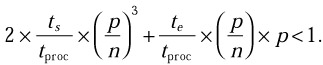So the algorithm would be scalable, if and only if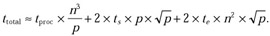that is, ifThis inequality will also be true only if p is small enough, whatever n and the ratio between p and n are. Therefore the improved algorithm is also not scalable on the network.

Further improvements of the algorithm cannot change the situation and result in a matrix-matrix multiplication algorithm that would be scalable on the network. The point is that there is nothing wrong with the algorithm. It is the network that has a problem, and the problem is its narrow bandwidth. The bandwidth puts limits on speedup and makes “normal” message-passing applications nonscalable on the network. In terms of scalability, this network is very similar to the SMP architecture, where the bandwidth of the memory bus is also a bottleneck limiting speedup (see Section 3.1).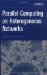Parallel Computing on Heterogeneous Networks (Wiley Series on Parallel and Distributed Computing)
ISBN: B000W7ZQBO
EAN: N/A
Year: 2005
Pages: 95